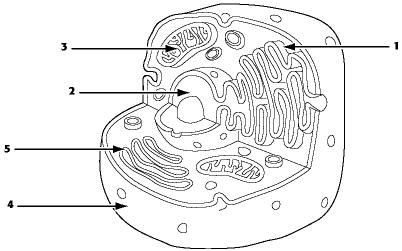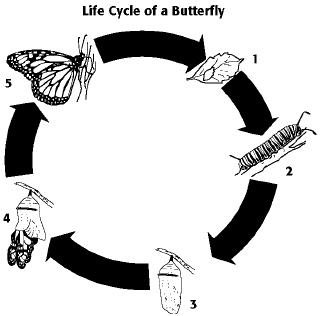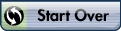Introduction, Chemistry, Water

%CODE1%

Javascript not enabled

Name:     ID:

Email:

Introduction, Chemistry, Water

Multiple Choice
Identify the choice that best completes the statement or answers the question.
1.
What is a localized group of organisms that belong to the same species?
 a. biosystem b. community c. population d. ecosystem e. organ system
2.
Plants convert the energy of sunlight into
 a. the energy of motion. b. carbon dioxide and water. c. the potential energy of chemical bonds. d. minerals. e. kinetic energy.
3.
As a result of photosynthesis, plants release ____ into the atmosphere.
 a. methane b. carbon dioxide c. water d. minerals e. oxygen
4.
Which of the following is the main source of energy for producers such as plants and other photosynthetic organisms?
 a. sunlight or solar energy b. carbon dioxide or kinetic energy c. heat or thermal energy d. chemicals or chemical energy e. both B and D
5.
The dynamics of any ecosystem include the following major processes:
 a. the flow of energy from sunlight to producers b. the flow of energy from sunlight to producers and then to consumers c. the recycling of chemical nutrients d. the flow of energy to producers and the recycling of nutrients e. the flow of energy from sunlight to producers and then to consumers, and the recycling of chemical nutrients
6.
What are the basic “building blocks” of deoxyribonucleic acid (DNA)?
 a. 100,000 different kinds of proteins b. 26 different kinds of chromosomes c. 20 different kinds of amino acids d. 4 different kinds of nucleotides e. 3 different kinds of genomes
7.
Which of the following is reflective of the phrase “the whole is greater than the sum of its parts”?
 a. high-throughput technology b. emergent properties c. natural selection d. reductionism e. feedback regulations
8.
Prokaryotic and eukaryotic cells generally have which of the following features in common?
 a. a membrane-bounded nucleus b. a cell wall made of cellulose c. ribosomes d. flagella or cilia that contain microtubules e. linear chromosomes made of DNA and protein
9.
Which of the following are characteristics shared by members of both domain Bacteria and domain Archaea?
 a. cytosol b. nucleus c. DNA d. A and C only e. A, B, and C
10.
A water sample from a hot thermal vent contained a single-celled organism that lacked a nucleus. What is its most likely classification?
 a. domain Eukarya b. domain Archaea c. kingdom Animalia d. kingdom Protista e. kingdom Fungi
11.
In a hypothetical world, every 50 years people over 6 feet tall are eliminated from the population. Based on your knowledge of natural selection, you would predict that the average height of the human population will
 a. remain unchanged. b. gradually decline. c. rapidly decline. d. gradually increase. e. rapidly increase.
12.
The method of scientific inquiry that describes natural structures and processes as accurately as possible through careful observation and the analysis of data is known as
 a. hypothesis-based science. b. discovery science. c. experimental science. d. quantitative science. e. qualitative science.
13.
What is a hypothesis?
 a. the same thing as an unproven theory b. a tentative explanation that can be tested and is falsifiable c. a verifiable observation sensed directly, or sensed indirectly with the aid of scientific instrumentation d. a fact based on qualitative data that is testable e. a fact based on quantitative data that is falsifiable
14.
The statement “If you show your dog affection, then your dog will seek your company” is an example of
 a. a statement that can be tested. b. a statement derived from a hypothesis. c. a prediction. d. deductive reasoning. e. all of the above
15.
Trace elements are those required by an organism in only minute quantities. Which of the following is a trace element that is required by humans and other vertebrates?
 a. nitrogen b. calcium c. iodine d. sodium e. phosphorus
16.
Which of the following statements is false?
 a. Atoms of the various elements differ in their number of subatomic particles. b. All atoms of a particular element have the same number of protons in their nuclei. c. The neutrons and protons present in the nucleus of an atom are almost identical in mass; each has a mass of about 1 dalton. d. An atom is the smallest unit of an element that still retains the properties of the element. e. Protons and electrons are electrically charged particles. Protons have one unit of negative charge, and electrons have one unit of positive charge.
17.
Each element is unique and different from other elements because of the number of protons in the nuclei of its atoms. Which of the following indicates the number of protons in an atom’s nucleus?
 a. atomic mass b. atomic weight c. atomic number d. mass weight e. mass number
18.
The mass number of an element can be easily approximated by adding together the number of ____ in an atom of that element.
 a. protons and neutrons b. energy levels c. protons and electrons d. neutrons and electrons e. isotopes
19.
The nucleus of a nitrogen atom contains 7 neutrons and 7 protons. Which of the following is a correct statement concerning nitrogen?
 a. The nitrogen atom has a mass number of approximately 7 daltons and an atomic mass of 14. b. The nitrogen atom has a mass number of approximately 14 daltons and an atomic mass of 7. c. The nitrogen atom has a mass number of 14 and an atomic mass of 7 grams. d. The nitrogen atom has a mass number of 7 grams and an atomic number of 14. e. The nitrogen atom has a mass number of 14 and an atomic mass of approximately 14 daltons.
20.
An atom with an atomic number of 9 and a mass number of 19 would have an atomic mass of approximately
 a. 9 daltons. b. 9 grams. c. 10 daltons. d. 20 grams. e. 19 daltons.
21.
Two isotopes of the same element will have different numbers of
 a. protons. b. neutrons. c. electrons. d. protons and neutrons. e. neutrons and electrons.
22.
Electrons exist only at fixed levels of potential energy. However, if an atom absorbs sufficient energy, a possible result is that
 a. an electron may move to an electron shell farther out from the nucleus. b. an electron may move to an electron shell closer to the nucleus. c. the atom may become a radioactive isotope. d. the atom would become a positively charged ion, or cation. e. the atom would become a negatively charged ion, or anion.
23.
The atomic number of neon is 10. Therefore, which of the following is correct about an atom of neon?
 a. It has 8 electrons in its outer electron shell. b. It is inert. c. It has an atomic mass of 10 daltons. d. A and B only e. A, B, and C are correct.
24.
From its atomic number of 15, it is possible to predict that the phosphorus atom has
 a. 15 neutrons. b. 15 protons. c. 15 electrons. d. 8 electrons in its outermost electron shell. e. B and C only
Use the figure below to answer the following questions.25.
Which drawing depicts the electron configuration of oxygen (O)?
 a. Drawing A b. Drawing B c. Drawing C d. Drawing D e. Drawing E
26.
Which drawing depicts an atom that is inert or chemically unreactive?
 a. Drawing A b. Drawing B c. Drawing C d. Drawing D e. Drawing E
27.
Which drawing depicts an atom with a valence of 2?
 a. Drawing A b. Drawing B c. Drawing C d. Drawing D e. Drawing E
Use the information extracted from the periodic table in the figure below to answer the following questions.28.
How many electrons does nitrogen have in its valence shell?
 a. 2 b. 5 c. 7 d. 8 e. 14
29.
How many neutrons are present in the nucleus of a phosphorus atom?
 a. 8 b. 15 c. 16 d. 31 e. 46
30.
How many electrons would be expected in the outermost electron shell of an atom with atomic number 12?
 a. 1 b. 2 c. 4 d. 6 e. 8
31.
What results from an unequal sharing of electrons between atoms?
 a. a nonpolar covalent bond b. a polar covalent bond c. an ionic bond d. a hydrogen bond e. a hydrophobic interaction
32.
A covalent bond is likely to be polar when
 a. one of the atoms sharing electrons is much more electronegative than the other atom. b. the two atoms sharing electrons are equally electronegative. c. the two atoms sharing electrons are of the same element. d. it is between two atoms that are both very strong electron acceptors. e. the two atoms sharing electrons are different elements.
Use the figure below to answer the following questions. .33.
What results from the chemical reaction?
 a. a cation with a net charge of +1 b. a cation with a net charge of -1 c. an anion with a net charge of +1 d. an anion with a net charge of -1 e. A and D
34.
What is the atomic number of the cation formed in the reaction?
 a. 1 b. 8 c. 10 d. 11 e. 16
35.
In ammonium chloride salt (NH4Cl) the anion is a single chloride ion, . What is the cation of NH4Cl?
 a. N, with a charge of +3 b. H, with a charge of +1 c. H2 with a charge of +4 d. NH4 with a charge of +1 e. NH4 with a charge of +4
36.
Van der Waals interactions result when
 a. hybrid orbitals overlap. b. electrons are not symmetrically distributed in a molecule. c. molecules held by ionic bonds react with water. d. two polar covalent bonds react. e. a hydrogen atom loses an electron.
37.
A van der Waals interaction is the weak attraction between
 a. the electrons of one molecule and the electrons of a nearby molecule. b. the nucleus of one molecule and the electrons of a nearby molecule. c. a polar molecule and a nearby nonpolar molecule. d. a polar molecule and a nearby molecule that is also polar. e. a nonpolar molecule and a nearby molecule that is also nonpolar.
38.
Which of the following is not considered to be a weak molecular interaction?
 a. a covalent bond b. a van der Waals interaction c. an ionic bond in the presence of water d. a hydrogen bond e. A and B only
39.
An example of a hydrogen bond is the bond between
 a. C and H in methane (CH4). b. the H of one water molecule and the O of another water molecule. c. Na+and Cl- in salt. d. the two hydrogen atoms in a molecule of hydrogen gas (H2). e. Mg+and Cl- in MgCl2.
40.
Which of the following is a correct definition of a kilocalorie?
 a. the amount of heat required to raise the temperature of 1 g of water by 1°F b. the amount of heat required to raise the temperature of 1 g of water by 1°C c. the amount of heat required to raise the temperature of 1 kg of water by 1°F d. the amount of heat required to raise the temperature of 1 kg of water by 1°C e. the amount of heat required to raise the temperature of 1,000 g of water by 1°F
41.
Desert rabbits are adapted to the warm climate because their large ears aid in the removal of heat due to the
 a. high surface tension of water. b. high heat of vaporization of water. c. high specific heat of water. d. buffering capacity of water. e. dissociation of water molecules.
42.
Temperature usually increases when water condenses. Which behavior of water is most directly responsible for this phenomenon?
 a. the change in density when it condenses to form a liquid or solid b. reactions with other atmospheric compounds c. the release of heat by the formation of hydrogen bonds d. the release of heat by the breaking of hydrogen bonds e. the high surface tension of water
43.
How many molecules of glucose (C6H2O6 molecular mass =180 daltons) would be present in one mole of glucose?
 a. 24 b. 342 c. 23 1014 d. 180 1014 e. 6.02 1023

Use the figure below to answer the following questions:44.
How many grams of the molecule in the figure above would be required to make 2.5 L of a 1 M solution of the molecule?
(Carbon = 12, Oxygen = 16, Hydrogen = 1)
 a. 29 b. 30 c. 60 d. 150 e. 342
45.
Which of the following ionizes completely in solution and is considered to be a strong base?
 a. NaCl b. HCl c. NH3 d. H2CO3 e. NaOH
46.
A given solution contains 0.0001(10-4) moles of hydrogen ions [H+] per liter. Which of the following best describes this solution?
 a. acidic: H+ acceptor b. basic: H+ acceptor c. acidic: H+ donor d. basic: H+ donor e. neutral
47.
A solution contains 0.0000001(10-7) moles of hydroxide ions [OH-] per liter. Which of the following best describes this solution?
 a. acidic: H+ acceptor b. basic: H+ acceptor c. acidic: H+ donor d. basic: H+ donor e. neutral
48.
Which of the following solutions has the greatest concentration of hydroxide ions [OH-]?
 a. lemon juice at pH 2 b. vinegar at pH 3 c. tomato juice at pH 4 d. urine at pH 6 e. seawater at pH 8
49.
Which of the following statements is true about buffer solutions?
 a. They maintain a constant pH when bases are added to them but not when acids are added to them. b. They maintain a constant pH when acids are added to them but not when bases are added to them. c. They maintain a constant pH of exactly 7 in all living cells and biological fluids. d. They maintain a relatively constant pH. e. They are found only in living systems and biological fluids.
50.

One of the buffers that contribute to pH stability in human blood is carbonic acid H2CO3. Carbonic acid is a weak acid that when placed in an aqueous solution dissociates into a bicarbonate ion (HCO3-) and a hydrogen ion (H+) Thus,

H2CO3 HCO3- + H+

If the pH of the blood increases, one would expect

 a. a decrease in the concentration of H2CO3 and an increase in the concentration of H2O. b. an increase in the concentration of H2CO3 and a decrease in the concentration of H2O. c. a decrease in the concentration of HCO3- and an increase in the concentration of H2O. d. an increase in the concentration of HCO3- and a decrease in the concentration of H2O. e. a decrease in the concentration of HCO3- and an increase in the concentration of both H2CO3and H2O.